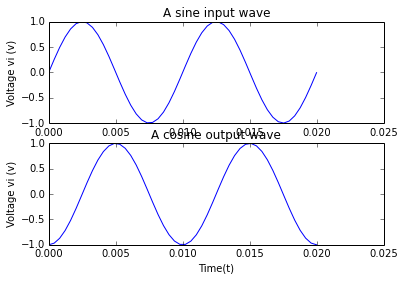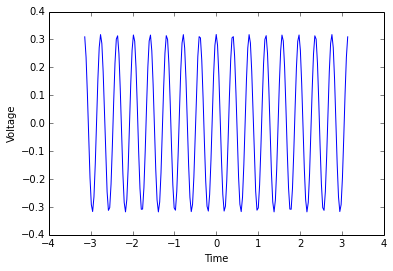# Chapter 4 : Operational Amplifier Applications¶

## Example 4.2 Page No.139¶

In :
# Given data
Rf = 50*10**3
R1 = 40*10**3
R2 = 25*10**3
R3 = 10*10**3
R4 = 20*10**3
R5 = 30*10**3

# Solution
R = (R1 * R2)/(R1 + R2)
Vo11 = (R + Rf)/R

def Vo(V1,V2,V3,V4):

return -1.25*V1 - 2.0*V2 + 2.32*V3 + 1.16*V4

Vo1 = Vo(2,3,4,5)

#Dispalying the output

print "The value of Vo = ",Vo1,"V"

The value of Vo =  6.58 V


## Example 4.3 Page No.167¶

In :
%matplotlib inline
import math
from pylab import figure, show
import numpy as np
# Given data

fmax = 100
Vp = 1

# solution part a

C1 = 0.1*10**-6 # Set it as the capacitance value.
Rf = 1/(2*math.pi*fmax*C1)
fb = 10*fmax
R1 = 1/(2*math.pi*fb*C1)
Cf = R1 *C1/Rf

# Solution part b
fig = figure(1)
t  = np.linspace(0,20*10**-3)
ax1.plot(t,np.sin(628*t))
ax1.set_ylabel('Voltage vi (v)')
ax1.set_xlabel('Time(t)')
ax1.set_title('A sine input wave')

ax2.plot(t,-np.cos(628*t))
ax2.set_ylabel('Voltage vi (v)')
ax2.set_xlabel('Time(t)')
ax2.set_title('A cosine output wave')

show()## Example 4.4 Page No.171¶

In :
%matplotlib inline
import matplotlib.pylab as plt
import numpy as np

# Given Data
R1 = 10*10**3
Rf = 100*10**3
Cf = 10*10**-9

# Solution - Finding the frequency limit of integration fa

fa = int(1/(2*np.pi*Rf*Cf))

print "The value of lower frequency limit of integration is =",fa,"Hz"

# Studying the response of sine wave input

V = 1
f = 5*10**3

vo = round(1/(R1*Cf*2*np.pi*5000),3)
x = np.linspace(-np.pi,np.pi,201)
plt.plot(x,vo*np.cos(2*np.pi*f*x))
plt.xlabel('Time')
plt.ylabel('Voltage')
plt.show()

The value of lower frequency limit of integration is = 159 Hz## Example 4.5 Page No.173¶

In :
import math
#Given data

w = 10000
Apeak = 20
A = 3
C = 0.1*10**-6

# Solution

Rf = math.sqrt(1/((10**-4)**2))
R1 = Rf/10

# Displaying the values

print "The value of Rf = ",int(Rf/10**3),"Kilo Ohms"
print "The value of R1 = ",int(R1/10**3),"Kilo Ohms"

The value of Rf =  10 Kilo Ohms
The value of R1 =  1 Kilo Ohms


## Example 4.9 Page No.178¶

In :
import numpy as np
# defining two functions

def x(t):

return 10*np.sin(3*t)

def x1(t):

return 30*np.cos(3*t)

print " The value of x(0) = ",int(x(0))
print " The value of defferential x(0) =",int(x1(0))

 The value of x(0) =  0
The value of defferential x(0) = 30

In [ ]: